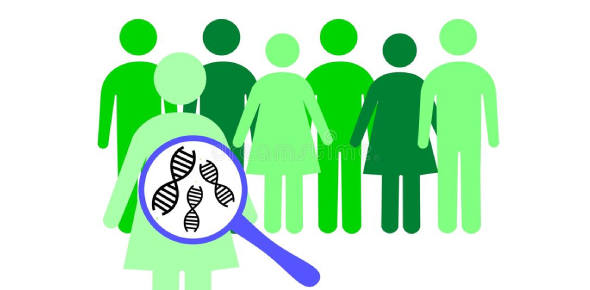# What Do You Know About Population Genetics? Trivia Quiz

5 Questions | Total Attempts: 1233SettingsWhat do you know about population genetics? Genetics is a wonderful study that lets us know more about our genetic makeup than the naked eye could ever allow us to. It makes us understand how likely we are to suffer from a life-threatening illness or have certain features that our parents have. Just how different is one person’s genetic makeup different from another, and why the change? Take the quiz to see how knowledgeable you are.

• 1.
If the frequency of the a allele is 0.1, then, if the population is at Hardy-Weinberg equilibrium, the percentage of individuals that are aa should be ______%
• 2.
If the frequency of the a allele is 0.1, then, if the population is at Hardy-Weinberg equilibrium, the percentage of individuals that are Aa should be ______%
• 3.
If the frequency of the a allele is 0.1, then, if the population is at Hardy-Weinberg equilibrium, the percentage of individuals that are AA should be ______%
• 4.
If, in a population at Hardy-Weinberg equilibrium, 4% of the individuals are aa, then the percentage of AA individual should be ______%
• 5.
Hardy-Weinberg equilibrium assumes that in the population.
• A.

No selection is occurring.

• B.

No mutation is occurring

• C.

No migration is occurring

• D.

Mating is random

• E.

The mating population is very large

• F.

All of the above

Related TopicsBack to top